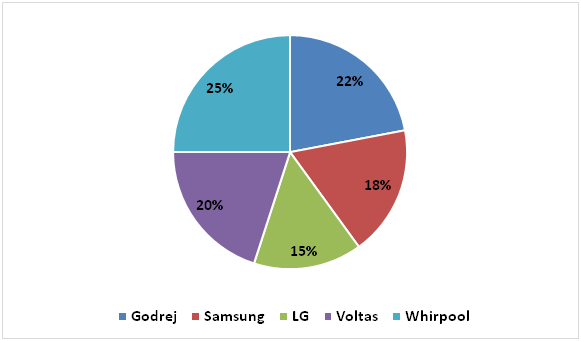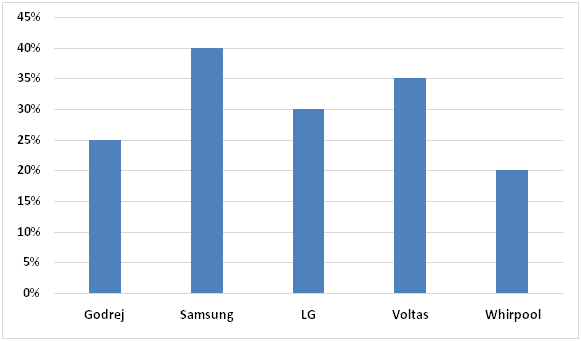# IBPS RRB Clerk Mains Quantitative Aptitude Questions 2019 (Day-02)

Dear Aspirants, Our IBPS Guide team is providing new series of Quantitative Aptitude Questions for IBPS RRB Clerk Mains 2019 so the aspirants can practice it on a daily basis. These questions are framed by our skilled experts after understanding your needs thoroughly. Aspirants can practice these new series questions daily to familiarize with the exact exam pattern and make your preparation effective.

Check here for IBPS RRB PO Mains Mock Test 2019

Check here for IBPS RRB Clerk Mains Mock Test 2019

Check here for IBPS PO Prelims Mock Test 2019

### Click Here to Subscribe Crack High Level Puzzles & Seating Arrangement Questions PDF 2019 Plan

[WpProQuiz 6937]

Directions (1 – 5): Study the following information carefully and answer the questions given below:

The following pie chart represents percentage wise distribution of number of refrigerators of five different companies sold by an electronic shop in 2017.

Total number of refrigerators of five different companies sold by the electronic shop in 2017 = 6000All the refrigerators are either of small size or big size. The following bar graph represents percentage of small size refrigerators among them.1) Find the respective ratio of the number of small size Godrej refrigerators sold by the shop in 2017 and number of big size LG refrigerators sold by the shop in that year.

a) 7:19

b) 9:17

c) 11:21

d) 13:19

e) None of these

2) Number of big size Voltas refrigerators sold by the shop in 2016 was 20% less than that of in 2017. Find the number of big size Voltas refrigerators sold by the shop in 2016.

a) 712

b) 548

c) 624

d) 728

e) None of these

3) Find the average of the number of small size Samsung refrigerators and number of small size LG refrigerators sold by the shop in 2017.

a) 351

b) 413

c) 381

d) 453

e) None of these

4) Number of Voltas refrigerators sold by the shop in 2016 was 25% more than that of in 2017 and out of them 35% are of small size. Find the number of big size Voltas refrigerators sold by the shop in 2016.

a) 685

b) 975

c) 855

d) 575

e) None of these

5) Number of big size refrigerators of which company sold by the shop in 2017 is maximum?

a) Godrej

b) Samsung

c) LG

d) Voltas

e) Whirlpool

Directions (6 – 10): In each of these questions two equations (I) and (II) are given. You have to solve both the equations and give answer,

6) I) 5x² – 16x + 11 = 0

II) 3y² + 19y + 30 = 0

a) If x > y

b) If x < y

c) If x ≥ y

d) If x ≤ y

e) If x = y or relation between x and y cannot be determined.

7) I) 2x²+3x – 20=0

II) 5y2 – 3y – 2 = 0

a) If x > y

b) If x < y

c) If x ≥ y

d) If x ≤ y

e) If x = y or relation between x and y cannot be determined.

8) I) 4x2 + 12x + 9 = 0

II) y² – 10y + 21 = 0

a) If x > y

b) If x < y

c) If x ≥ y

d) If x ≤ y

e) If x = y or relation between x and y cannot be determined.

9) I) 3x² + 12x + 9 = 0

II) 2y2 – 7y + 6 = 0

a) If x > y

b) If x < y

c) If x ≥ y

d) If x ≤ y

e) If x = y or relation between x and y cannot be determined.

10) I) x2 – 52x + 667 = 0

II) 2y2 + 20y + 50 = 0

a) If x > y

b) If x < y

c) If x ≥ y

d) If x ≤ y

e) If x = y or relation between x and y cannot be determined.

Direction (1-5) :

Number of small size Godrej refrigerators sold by the shop in 2017

= 25/100 x 22/100 x 6000

= 330

Number of big size LG refrigerators sold by the shop in 2017

= (100 – 30)/100 x 15/100 x 6000

= 70/100 x 15/100 x 6000

= 630

Required ratio = 330 : 630

= 11:21

Number of big size Voltas refrigerators sold by the shop in 2017

= (100 – 35)/100 x 20/100 x 6000

= 65/100 x 20/100 x 6000

= 780

Number of big size Voltas refrigerators sold by the shop in 2016 = 780 x 80/100

= 624

Number of small size Samsung refrigerators sold by the shop in 2017 = 40/100 x 18/100 x 6000

= 432

Number of small size LG refrigerators sold by the shop in 2017 = 30/100 x 15/100 x 6000

= 270

Required average = (432 + 270)/2

= 351

Number of Voltas refrigerators sold by the shop in 2016 = 20/100 x 6000 x 125/100

= 1500

Number of big size Voltas refrigerators sold by the shop in 2016 = (100 – 35)/100 x 1500

= 65/100 x 1500

= 975

Number of big size Godrej refrigerators sold by the shop in 2017

= (100 – 25)/100 x 22/100 x 6000

= 75/100 x 22/100 x 6000

= 990

Number of big size Samsung refrigerators sold by the shop in 2017

= (100 – 40)/100 x 18/100 x 6000

= 60/100 x 18/100 x 6000

= 648

Number of big size LG refrigerators sold by the shop in 2017

= (100 – 30)/100 x 15/100 x 6000

= 70/100 x 15/100 x 6000

= 630

Number of big size Voltas refrigerators sold by the shop in 2017

= (100 – 35)/100 x 20/100 x 6000

= 65/100 x 20/100 x 6000

= 780

Number of big size Whirlpool refrigerators sold by the shop in 2017

= (100 – 20)/100 x 25/100 x 6000

= 80/100 x 25/100 x 6000

= 1200 (Maximum)

Directions (6 – 10):

I) 5x² – 16x + 11 = 0

=> 5x2 – 5x – 11x + 11 = 0

=> 5x(x – 1) – 11(x – 1) = 0

=> (5x – 11)(x – 1) = 0

=> x = 11/5, 1

II) 3y² + 19y + 30 = 0

=> 3y2 + 9y + 10y + 30 = 0

=> 3y(y + 3) + 10(y + 3) = 0

=> (3y + 10)(y + 3) = 0

=> y = -10/3, -3

Hence, x > y

I) 2x²+3x – 20=0

=> 2x2 + 8x – 5x – 20 = 0

=> 2x(x + 4) – 5(x + 4) = 0

=> (2x – 5)(x + 4) = 0

=> x = 5/2, -4

II) 5y2 – 3y – 2 = 0

=> 5y2 – 5y + 2y – 2 = 0

=> 5y(y – 1) + 2(y – 1) = 0

=> (5y + 2)(y – 1) = 0

=> y = -2/5, 1

Hence, relation between x and y cannot be determined.

I) 4x2 + 12x + 9 = 0

=> 4x2 + 6x + 6x + 9 = 0

=>2x(2x + 3) + 3(2x + 3) = 0

=> (2x + 3)(2x + 3) = 0

=> x = -3/2, -3/2

II) y² – 10y + 21 = 0

=> y2 – 3y – 7y + 21 = 0

=>y(y – 3) – 7(y – 3) = 0

=> (y – 7)(y – 3) = 0

=> y = 7, 3

Hence, x < y

I) 3x² + 12x + 9 = 0

=> 3x2 + 3x + 9x + 9 = 0

=> 3x(x + 1) + 9(x + 1) = 0

=> (3x + 9)(x + 1) = 0

=> x = -3, -1

II) 2y2 – 7y + 6 = 0

=> 2y2 – 3y – 4y + 6 = 0

=>y(2y – 3) – 2(2y – 3) = 0

=> (y – 2)(2y – 3) = 0

=> y = 2, 3/2

Hence, x < y

I) x2 – 52x + 667 = 0

=>x2 – 23x – 29x + 667 = 0

=>x(x – 23) – 29(x – 23) = 0

=> (x – 29)(x – 23) = 0

=> x = 29, 23

II) 2y2 + 20y + 50 = 0

=> 2y2 + 10y + 10y + 50 = 0

=> 2y(y + 5) + 10(y + 5) = 0

=> (2y + 10)(y + 5) = 0

=> y = -5, -5

Hence, x > y

5 1 vote
Rating